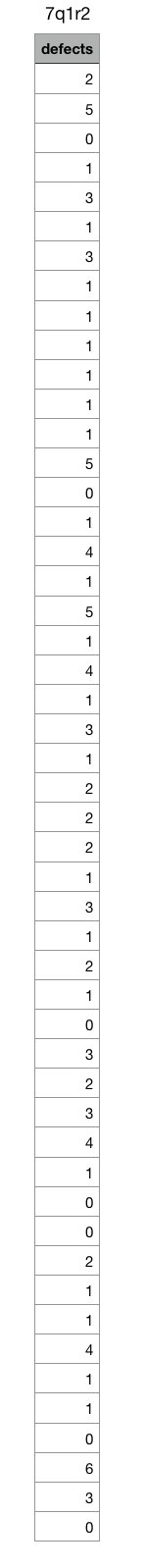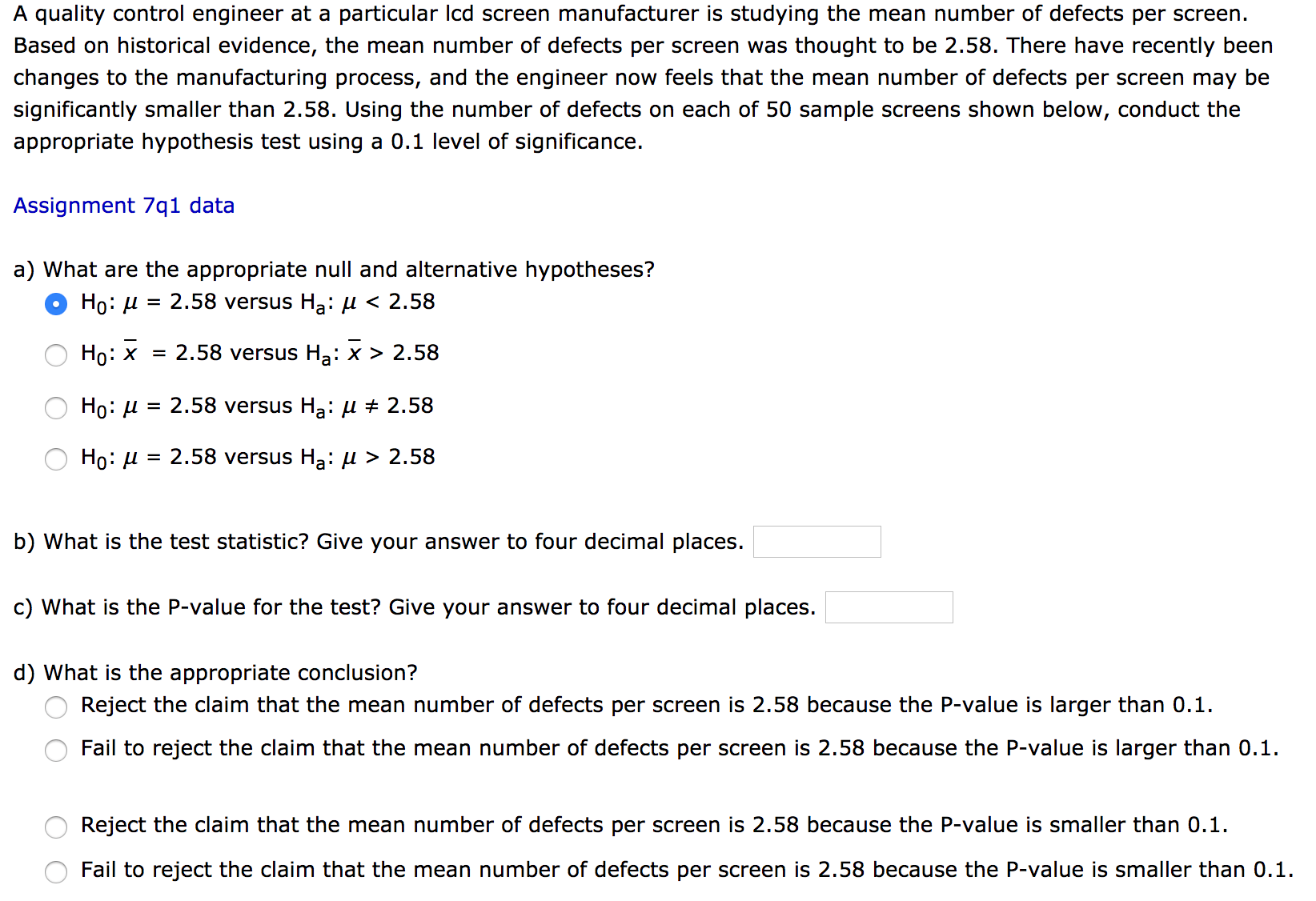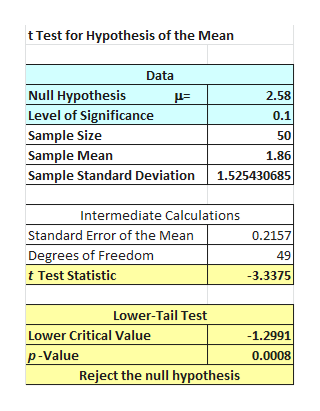# 7q1r2defects250131311115014151413122213121023100211110630 A quality control engineer at a particular Icd screen manufacturer is studying the mean number of defects per screen.Based on historical evidence, the mean number of defects per screen was thought to be 2.58. There have recently beenchanges to the manufacturing process, and the engineer now feels that the mean number of defects per screen may besignificantly smaller than 2.58. Using the number of defects on each of 50 sample screens shown below, conduct theappropriate hypothesis test using a 0.1 level of significance.Assignment 7q1 dataa) What are the appropriate null and alternative hypotheses?2.58 versus Ha: u < 2.58Ho:Но: х= 2.58 versus Ha: x > 2.58Но: и= 2.58 versus Ha: u # 2.58Но: и %3D 2.58 versus Ha: И> 2.58b) What is the test statistic? Give your answer to four decimal places.c) What is the P-value for the test? Give your answer to four decimal places.d) What is the appropriate conclusion?Reject the claim that the mean number of defects per screen is 2.58 because the P-value is larger than 0.1.Fail to reject the claim that the mean number of defects per screen is 2.58 because the P-value is larger than 0.1.Reject the claim that the mean number of defects per screen is 2.58 because the P-value is smaller than 0.1.Fail to reject the claim that the mean number of defects per screen is 2.58 because the P-value is smaller than 0.1

Question
18 viewshelp_outlineImage Transcriptionclose7q1r2 defects 2 5 0 1 3 1 3 1 1 1 1 5 0 1 4 1 5 1 4 1 3 1 2 2 2 1 3 1 2 1 0 2 3 1 0 0 2 1 1 1 1 0 6 3 0 fullscreenhelp_outlineImage TranscriptioncloseA quality control engineer at a particular Icd screen manufacturer is studying the mean number of defects per screen. Based on historical evidence, the mean number of defects per screen was thought to be 2.58. There have recently been changes to the manufacturing process, and the engineer now feels that the mean number of defects per screen may be significantly smaller than 2.58. Using the number of defects on each of 50 sample screens shown below, conduct the appropriate hypothesis test using a 0.1 level of significance. Assignment 7q1 data a) What are the appropriate null and alternative hypotheses? 2.58 versus Ha: u < 2.58 Ho: Но: х = 2.58 versus Ha: x > 2.58 Но: и = 2.58 versus Ha: u # 2.58 Но: и %3D 2.58 versus Ha: И> 2.58 b) What is the test statistic? Give your answer to four decimal places. c) What is the P-value for the test? Give your answer to four decimal places. d) What is the appropriate conclusion? Reject the claim that the mean number of defects per screen is 2.58 because the P-value is larger than 0.1. Fail to reject the claim that the mean number of defects per screen is 2.58 because the P-value is larger than 0.1. Reject the claim that the mean number of defects per screen is 2.58 because the P-value is smaller than 0.1. Fail to reject the claim that the mean number of defects per screen is 2.58 because the P-value is smaller than 0.1 fullscreen
check_circle

Step 1

a)

The hypotheses for the test are stated below:

From the information, the level of significance is 0.10 and the claim of the test is the mean number of defects per screen may be significantly smaller than 2.58.

The claim of the test is there has been no change in the mean length.

Null hypothesis:

H0: µ = 2.58

Alternative hypothesis:

Ha: µ < 2.58

The correct answer is H0: µ = 2.58 versus Ha: µ < 2.58.

b)

The test statistics is obtained below:

Instructions to obtain the p-value for t test are listed:

1. In EXCEL, Select Add-Ins > PHStat > One-Sample Tests > t Test for the mean, Sigma unknown.
2. Enter 58 under Null hypothesis.
3. Enter 10 under Level of significance.
4. In Sample Statistic Options, choose Sample Statistic unknown \$A1\$A51.
5. In Test Options, choose Two (or Upper or Lower) Tail Test.
6. In Output Options, enter a Title and click OK.

Follow the above instructions to obtain the below output:help_outlineImage Transcriptioncloset Test for Hypothesis of the Mean Data Null Hypothesis 2.58 Level of Significance 0.1 Sample Size Sample Mean Sample Standard Deviation 50 1.86 1.525430685 Intermediate Calculations 0.2157 Standard Error of the Mean Degrees of Freedom 49 t Test Statistic -3.3375 Lower-Tail Test -1.2991 Lower Critical Value p-Value 0.0008 Reject the null hypothesis fullscreen
Step 2

From MegaStat output, the test statistics is ­−3.3375.

Therefore, the test statistics is −3.3375.

c)

From MegaStat output, the ...

### Want to see the full answer?

See Solution

#### Want to see this answer and more?

Solutions are written by subject experts who are available 24/7. Questions are typically answered within 1 hour.*

See Solution
*Response times may vary by subject and question.
Tagged in

### Statistics